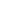List price of a book is Rs.100. A dealer sells three such books for Rs.274.50 after allowing discount at a certain rate. Find the rate of discount

a) 8.16%
b) 8.5%
c) 8.34 %
d) 8.75 %
SSC CGL-2015

 8.5%. Solution Marked price = Rs 100 Marked price of 3 books = Rs. 300 Selling price of 3 books = Rs. 274.50 Discount = 300 - 274.5 = 25.5 Discount on Rs.300 = 25.5 Discount on Rs.100 = 25.5 x 100 /300 = 8.5 So the rate of discount is 8.5%
Share :
+
Next
« Prev
Prev
Next »